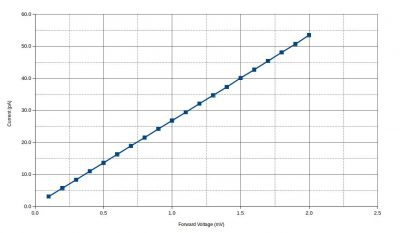## Diode Equation and Forward Current Measurement

We are all familiar with the I/V characteristics of a diode. The main characteristics of a typical diode is that when forward biased, it starts to conduct as the voltage applied approaches the threshold voltage of the diode and the current increases exponentially as the forward voltage increases. This behavior can be modeled by the Shockley diode equation. A question frequently asked is that what happens when the applied forward voltage is very small, say just a few millivolts?

According to the diode equation, the current flowing through the diode is determined by:
$I_D = I_S\left(e^{\frac{V_D}{nV_T}}-1\right)$

where Vd is the forward voltage applied between the anode and cathode of the diode, Is is the reverse saturation current – a constant, n is the emission coefficient – again a constant, and Vt is the thermal voltage which is roughly 26mV under room temperature. So when the forward is in the millivolt range, the exponent is a small positive number. Because of this, we can use the first order Taylor expansion to approximate the term
$e^{\frac{V_D}{nV_T}} \approx 1 + \frac{V_D}{nV_T} \quad\quad where \quad V_D << 1mV$ So the forward current becomes a linear function of the forward voltage applied: $I_D = \beta V_D \quad\quad where \quad \beta= \frac{I_S}{nV_T}$ And this is the I/V characteristic we'd expect when the forward voltage Vd is small. As an experiment to illustrate this behavior, I used my Keithley 614 electrometer along with a EDC/Krohn-Hite MV216A voltage standard and measured the forward current of an 1N4004 rectifier diode when the forward biasing voltage was increased from 0V to 2mV in 0.1mV step. The results are shown below, note the the current is in picoampere.

V (mV), I (pA)
0.1, 3.1
0.2, 5.7
0.3, 8.3
0.4, 11.0
0.5, 13.6
0.6, 16.3
0.7, 18.9
0.8, 21.5
0.9, 24.2
1.0, 26.8
1.1, 29.4
1.2, 32.1
1.3, 34.7
1.4, 37.3
1.5, 40.1
1.6, 42.7
1.7, 45.4
1.8, 48.1
1.9, 50.7
2.0, 53.5

And here is the plotted measurement data:So indeed, the measured I/V curve is approximately linear within this forward voltage range. This behavior will continue for a while until the forward voltage increases near the conducting threshold and then the exponential nature of the curve will become more prominent.

The video below discusses the experiment and the setup in detail:

1.jluu says:
•kwong says: